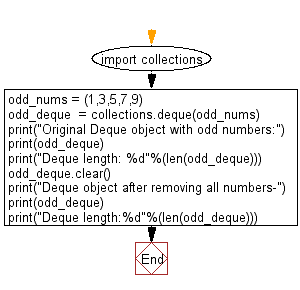﻿ Python: Remove all the elements of a given deque object - w3resource# Python: Remove all the elements of a given deque object

## Python Collections: Exercise-10 with Solution

Write a Python program to remove all the elements of a given deque object.

Sample Solution:

Python Code:

``````import collections
odd_nums = (1,3,5,7,9)
odd_deque  = collections.deque(odd_nums)
print("Original Deque object with odd numbers:")
print(odd_deque)
print("Deque length: %d"%(len(odd_deque)))
odd_deque.clear()
print("Deque object after removing all numbers-")
print(odd_deque)
print("Deque length:%d"%(len(odd_deque)))
```
```

Sample Output:

```Original Deque object with odd numbers:
deque([1, 3, 5, 7, 9])
Deque length: 5
Deque object after removing all numbers-
deque([])
Deque length:0
```

Flowchart:## Visualize Python code execution:

The following tool visualize what the computer is doing step-by-step as it executes the said program:

Python Code Editor:

Have another way to solve this solution? Contribute your code (and comments) through Disqus.

What is the difficulty level of this exercise?

Test your Programming skills with w3resource's quiz.

﻿

## Python: Tips of the Day

Use Reversed() In for Loops:

```>>> tasks = ['laundry', 'picking up kids', 'gardening', 'cooking']